### Iterative Algorithms for Model Fitting

Two iterative maximum likelihood algorithms are available in PROC SURVEYLOGISTIC  to obtain the pseudo-estimate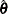of the model parameter. The default is the Fisher scoring method, which is equivalent to fitting by iteratively reweighted least squares. The alternative algorithm is the Newton-Raphson method. Both algorithms give the same parameter estimates; the covariance matrix ofis estimated in the section Variance Estimation. For a generalized logit model, only the Newton-Raphson technique is available. You can use the TECHNIQUE= option in the MODEL statement to select a fitting algorithm.

#### Iteratively Reweighted Least Squares Algorithm (Fisher Scoring)

Letbe the response variable that takes values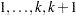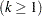. Letindex all observations and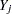be the value of response for theth observation. Consider the multinomial variable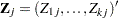such that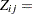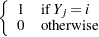and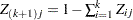. With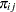denoting the probability that the jth observation has response value i, the expected value of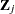is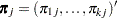, and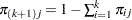. The covariance matrix ofis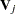, which is the covariance matrix of a multinomial random variable for one trial with parameter vector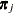. Letbe the vector of regression parameters—for example,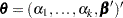for cumulative logit model. Let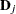be the matrix of partial derivatives ofwith respect to. The estimating equation for the regression parameters is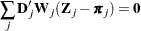where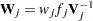, and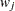and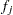are the WEIGHT and FREQ values of theth observation.

With a starting value of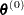, the pseudo-estimate ofis obtained iteratively as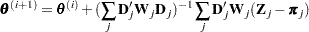where,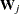, andare evaluated at theth iteration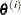. The expression after the plus sign is the step size. If the log likelihood evaluated at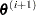is less than that evaluated at, thenis recomputed by step-halving or ridging. The iterative scheme continues until convergence is obtained—that is, untilis sufficiently close to. Then the maximum likelihood estimate ofis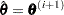.

By default, starting values are zero for the slope parameters, and starting values are the observed cumulative logits (that is, logits of the observed cumulative proportions of response) for the intercept parameters. Alternatively, the starting values can be specified with the INEST= option in the PROC SURVEYLOGISTIC statement.

#### Newton-Raphson Algorithm

Let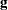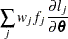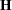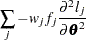be the gradient vector and the Hessian matrix, where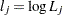is the log likelihood for theth observation. With a starting value of, the pseudo-estimateofis obtained iteratively until convergence is obtained: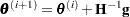where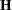andare evaluated at theth iteration. If the log likelihood evaluated atis less than that evaluated at, thenis recomputed by step-halving or ridging. The iterative scheme continues until convergence is obtained—that is, untilis sufficiently close to. Then the maximum likelihood estimate ofis.